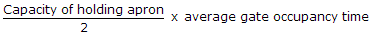# Civil Engineering - UPSC Civil Service Exam Questions

36.

For stream function ψ = 3x2 - y3, the magnitude of velocity at the point (2, 1) is

 A. 12.37 B. 12 C. 13 D. 13.5

Explanation:

No answer description available for this question. Let us discuss.

37.

Consider the following statements about provision of sag rods :
1. Sag rods reduce span length of purlin in the weak direction.
2. Sag rods are installed in the plane of the roof.
3. Ridge purlin is subject to vertical components of sag rods on either side of slope.
Which of the statements given above are correct ?

 A. 1, 2 and 3 B. 2 and 3 C. 1 and 2 D. 1 and 3

Explanation:

No answer description available for this question. Let us discuss.

38.

The smallest scale adopted for topographical surveys is

 A. 1 : 25, 000 B. 1 : 50, 000 C. 1 : 2, 50, 000 D. 1 : 5, 00, 000

Explanation:

No answer description available for this question. Let us discuss.

39.

A shot of mass m penetrates a thickness t of a fixed plate of mass M. If the plate were free to move and the resistance supposed to be uniform, which one of the following is the thickness penetrated ?

 A.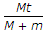B.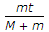C.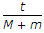D.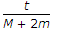Explanation:

No answer description available for this question. Let us discuss.

40.

Which one of the following gives the number of gate position in an airport ?

 A.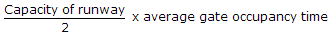B.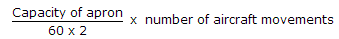C.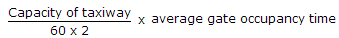D.